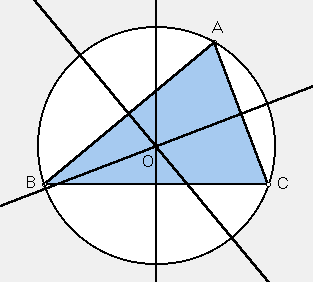# CIRCUMCENTER

 In the plane of any triangle ABC, let D = the perpendicular bisector of side BC, E = the perpendicular bisector of side CA, F = the perpendicular bisector of side AB. As you see in the sketch, the three perpendicular bisectors all come together in one point, called the circumcenter of triangle ABC. The circumcenter is equidistant from the three vertices, and so the common distance is the radius of a circle that passes through the vertices. It is called the circumcircle.Triangle Centers